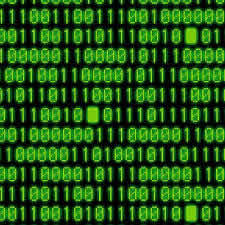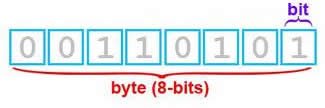# Difference Between Bits and Bytes

##### Key Difference: A bit is the smallest unit of data in a computer, whereas a byte is a unit of data that is composed of eight bits that are arranged sequentially.

A bit is the smallest unit of data in a computer, it is a single binary digit; it means that the digit can have either of two values and the two values are 0 and 1. Bits are used to encode a single unit of digital information. Computers operate on this base 2 number system. Computer uses bits as bits can easily be implemented with current electronic technology. Bit is basically a short name for binary digit.A binary number can either hold 0 or 1. For example, 1010 is a binary number. But to find out the value of binary number, a base of 2 is used. Zero represents off state and one represent on state and the computer can communicate only through these two states. When electricity flows into a transistor of a computer, in order to turn it on, it represents the value 1 and when the computer turns off a transistor it can represent 0. We can also define one bit as the smallest possible measurement of any kind of data.

1010 can be expressed as:

(1*2^3) + (0*2^2) + (1*2^1) + (0*2^0) = 8 + 0 + 2=10

Thus, 1010 as a binary number represents value of 10. And the number of bits in this binary number is four. A bit can be stored by a digital device or any other physical system, but it must fulfill the condition to exist in either of two possible distinct states

According to Wikipedia, the term byte was coined by Werner Buchholz in July 1956, during the early design phase for the IBM Stretch computer. A byte is a collection of eight bits. The eight bits can be in any order like – it may be 00000000 or 11111111 or 01110110 or any other possible ordering of 0 and 1. A byte can therefore represent values ranging from 0 to 255. These bytes are used to represent letters of the alphabet and other characters. This assignment is a part of the standard for converting text to binary known as ASCII (or ASCII code). It means that every letter that is read on the computer screen is made up of one byte, or eight bits. A byte is also referred as an octet.

The relation between the bits and bytes is quite obvious, as a byte is a collection of eight bits. Thus, bytes would have not existed without bits.

Image Courtesy: the-tech-guy.net, meesterbrein.com